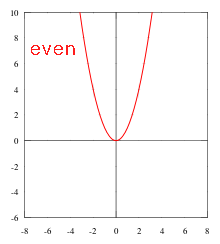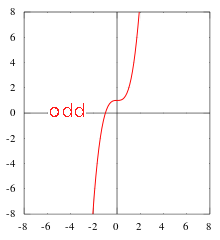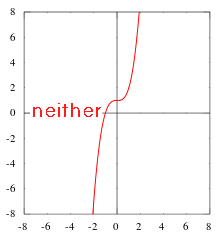Uh Oh! It seems you’re using an Ad blocker!

Since we’ve struggled a lot to makes online calculations for you, we are appealing to you to grant us by disabling the Ad blocker for this domain.# Even or Odd Function Calculator

Function F(x) = or Y = :Variable:

Table of Content

 1 What is an Even, Odd, or Neither Function? 2 How to Know if a Function is Even or Odd or Neither? 3 Set Representation of Odd and Even Numbers: 4 Is cos X an odd function? 5 Is tan an even or odd function? 6 Why Zero is even number?

Get the Widget!

Add this calculator to your site and lets users to perform easy calculations.

Feedback

How easy was it to use our calculator? Did you face any problem, tell us!

An online even or odd function calculator will help you to identify a certain function is even, odd, or neither function. Usually, the sign of values in the function did not matter during the calculation of function values, and only half values in the domain will be used. In this article, we will look at the definitions, properties, and how to find if a function is even or odd.

## What is an Even, Odd, or Neither Function?

Even Function:

A function f(x) is even, when f (x) = f (-x) for all values of x. It means that the function f (x) is the same for the positive and negative x-axis, or graphically, symmetric about the y-axis:
For example:

The function f (x) = x^2Properties of Even Function:

• The sum of even functions is even.
• The difference of even functions is even.
• Product of even functions is even.
• The quotient of even function is even.
• The composition of functions is even.
• The composition of an even and an odd function is even.

If required, you can verify all the above properties with an odd or even function calculator.

However, an online Inverse Function Calculator will allow you to determine the inverse of any given function with comprehensive calculations.

Odd Function:

A function f (x) is odd, when f (- x) = – f (x), for all x in the given function. So, the sign is inverted from one side of the x-axis to the other side. However, an online even or odd function calculator uses the same concept to identify if a function is odd or even.
Now, look at the graph of a function f (x) = x^3Properties of Odd function:

• The sum of odd functions is odd.
• The difference of odd functions is odd.
• The product of odd functions is even.
• The quotient of odd function is even.
• The composition of odd functions is odd.
• The composition of an odd function and an even function is even.

Neither Odd Nor Even function:

If a function does not express symmetry, then the function can be neither odd nor even. Therefore, an online even odd or neither calculator is able to determine whether a function is odd or even. For example, x^3 + 1 is neither function.### How to Know if a Function is Even or Odd or Neither?

You may be asked to find algebraically whether the function is odd, even, or neither.
For Even Function:
If we put (- x) into the function f(x) and get the starting or original function again, then this shows that f(x) is an even function.
$$f (x) → f (-x) = f (x)$$
Example:
Determine the algebraically function even odd or neither.
$$f (x) = 2x^2 – 3$$
Solution:
Well, you can use an online odd or even function calculator to check whether a function is even, odd or neither. For this purpose, it substitutes – x in the given function $$f (x) = 2x^2 – 3$$ and then simplifies.
$$f (x) = 2x^2 – 3$$
Now, plug in – x in the function,
$$f (- x) = 2(- x)^2 – 3$$
$$f (- x) = 2(- 1x)^2 – 3$$
$$f (- x) = 2 (- 1)^2 (x)^2 – 3$$
$$f (- x) = 2 (1) (x^2) – 3$$
$$f (- x) = 2x^2 – 3$$
Hence, f (- x) = f (x), which means if we substitute the same values in an online even or odd calculator, it displays the same results that are even function.

However, an online Composite Function Calculator can help you to evaluate the composition of the functions from entered values of functions f(x) and g(x) at the specific points.
For Odd Function:
If we substitute (- x) into the function f (x) and obtain the opposite or negative value of the function, then this implies that function f (x) is an odd function.
$$f (x) → f (-x) = – f (x)$$
Example:
How to determine even or odd function when,
$$f (x) = – x^7 + 8x^5 – x^3 + 6x$$
Solution:
Given function:
$$f (x) = – x^7 + 8x^5 – x^3 + 6x$$
Now, substitute – x into the given function f (x) and simplifying,
$$f (- x) = – (- x)^7 + 8(- x)^5 – (- x)^3 + 6(- x)$$
$$f (- x) = – (- 1)^7 (x)^7 + 8 (- 1)^5 (x)^5 – (- 1)^3 (x)^3 + 6 (- 1) (x)$$
$$f (- x) = – (- 1) x^7 + 8 (- 1) x^5 – (- 1) x^3 – 6 (x)$$
$$f (- x) = x^7 – 8 x^5 + x^3 – 6x$$
If you use an even or odd function calculator, then it shows the obtained function is not a starting function. Therefore, it is not an even function.
$$f (- x) = -1 (x^7 + 8 x^5 – x^3 + 6x)$$
Factor out the -1
$$f (- x) = – (x^7 + 8 x^5 – x^3 + 6x)$$
Hence,
$$f (- x) = – f (x)$$
After factoring out the -1 the function is equal to the starting function, which shows it is an odd function.
For Neither Function:
If plugin the (- x) into the function f (x) and we don’t get either even or odd, then that implies the given function f (x) is neither odd nor even function. In simple words, it does not fall under the classification of being odd or even.
$$f (- x) ≠ – f (x) And f (- x) ≠ f (x)$$
Example:
How to tell about even odd or neither functions, when:
$$f (x) = x^3 – x^2 – 1$$
Solution:
Given function:
$$f (x) = x^3 – x^2 – 1$$
Now, add – x in the given function.
$$f (- x) = (- x)^3 – (- x)^2 – 1$$
$$f (- x) = (- 1)^3 (x)^3 – (- 1)^2 (x)^2 – 1$$
So,
$$f (- x) = (- 1) x^3 – (1) x^2 – 1$$
$$f (- x) = – x^3 – x^2 – 1$$
Here,
f (- x) ≠ f (x) not an even function.
Also,
$$f (- x) = – (x^3 + x^2 + 1)$$
Which is not an odd function.
Therefore, the function f (x) is neither odd nor even.

### Set Representation of Odd and Even Numbers:

The sets of odd and even number can be represented as:

$$Odd = {2x + 1 : x ϵ Z}$$

$$Even = {2x : x ϵ Z}$$

A formal definition of an odd number is an integer of the form n = 2x + 1, where x is an integer. An even number is defined as an integer of the form n = 2x. This type of classification applies only to integers. Non-integer numbers like 3.462, 7/9, or infinity are neither odd nor even.

## How does Even or Odd Function Calculator Works?

An online even odd or neither calculator determine whether the function is odd, even, or neither by the following steps:

### Input:

• First, enter a given function and select the variable from the drop-down list.
• Hit the “Calculate” button.

### Output:

• The odd or even function calculator displays the nature of the function as even, odd, or neither.

## FAQs:

### Is cos X an odd function?

Cosine is an even function and sin is an odd function. You may not come across these adjectives even and odd when applied to the functions, but it’s important to know them.

### Is tan an even or odd function?

Sin, cos, and tan are trigonometric functions, they can be expressed as odd or even functions as well. Tangent and sine are both odd functions, and cos is an even function. Mathematically, we can define it as
Tan (-x) = – tan x
Cos (-x) = cos x
Sin (-x) = -sin x

### Why Zero is even number?

Zero is an integer multiply of 2 such as 0 x 2, due to this reason we can ask zero is an even number.

## Conclusion:

You may ask to find algebraically whether the function is odd or even. For this purpose, use our online even or odd function calculator that simplifies the entered function quickly without any hesitation. While looking at the function that needed to be graphed for assignment, a student or tutor can recognize with our calculator that would go quickly because the signed values do not matter in the calculations of function values.

## Reference:

From the source of Wikipedia: Even functions, Odd functions, Uniqueness, Addition and subtraction, multiplication and division, Composition, Even–odd decomposition.

From the source of Lumen Learning: Determine whether a function is even, odd, or neither from its graph, Set Representation of Even and Odd Numbers, Properties of Even and Odd Numbers, Even and Odd Decimals.

From the source of Libre Text: Odd and Even Functions, Types of Functions: Even, Odd, or Neither, Set Representation of Even and Odd Numbers, Neither Odd nor Even.